# The SURVEYLOGISTIC Procedure

### MODEL Statement

Subsections:
• MODEL events/trials = <effects </ options>>;

• MODEL variable <(v-options)> = <effects> </ options>;

The MODEL statement names the response variable and the explanatory effects, including covariates, main effects, interactions, and nested effects; see the section Specification of Effects in Chapter 45: The GLM Procedure, for more information. If you omit the explanatory variables, the procedure fits an intercept-only model. Model options can be specified after a slash (/).

Two forms of the MODEL statement can be specified. The first form, referred to as single-trial syntax, is applicable to binary, ordinal, and nominal response data. The second form, referred to as events/trials syntax, is restricted to the case of binary response data. The single-trial syntax is used when each observation in the DATA= data set contains information about only a single trial, such as a single subject in an experiment. When each observation contains information about multiple binary-response trials, such as the counts of the number of subjects observed and the number responding, then events/trials syntax can be used.

In the events/trials syntax, you specify two variables that contain count data for a binomial experiment. These two variables are separated by a slash. The value of the first variable, events, is the number of positive responses (or events), and it must be nonnegative. The value of the second variable, trials, is the number of trials, and it must not be less than the value of events.

In the single-trial syntax, you specify one variable (on the left side of the equal sign) as the response variable. This variable can be character or numeric. Options specific to the response variable can be specified immediately after the response variable with parentheses around them.

For both forms of the MODEL statement, explanatory effects follow the equal sign. Variables can be either continuous or classification variables. Classification variables can be character or numeric, and they must be declared in the CLASS statement. When an effect is a classification variable, the procedure enters a set of coded columns into the design matrix instead of directly entering a single column containing the values of the variable.

#### Response Variable Options

You specify the following options by enclosing them in parentheses after the response variable:

DESCENDING
DESC

reverses the order of response categories. If both the DESCENDING and the ORDER= options are specified, PROC SURVEYLOGISTIC orders the response categories according to the ORDER= option and then reverses that order. See the section Response Level Ordering for more detail.

EVENT=’category’ | keyword

specifies the event category for the binary response model. PROC SURVEYLOGISTIC models the probability of the event category. The EVENT= option has no effect when there are more than two response categories. You can specify the value (formatted if a format is applied) of the event category in quotes or you can specify one of the following keywords. The default is EVENT=FIRST.

FIRST

designates the first ordered category as the event

LAST

designates the last ordered category as the event

One of the most common sets of response levels is {0,1}, with 1 representing the event for which the probability is to be modeled. Consider the example where Y takes the values 1 and 0 for event and nonevent, respectively, and Exposure is the explanatory variable. To specify the value 1 as the event category, use the following MODEL statement:

   model Y(event='1') = Exposure;

ORDER=DATA | FORMATTED | FREQ | INTERNAL

specifies the sort order for the levels of the response variable. By default, ORDER=INTERNAL. For ORDER=FORMATTED and ORDER=INTERNAL, the sort order is machine-dependent.

When the default ORDER=FORMATTED is in effect for numeric variables for which you have supplied no explicit format,

Value of ORDER=

Levels Sorted By

DATA

Order of appearance in the input data set

FORMATTED

External formatted value, except for numeric

variables with no explicit format, which are

sorted by their unformatted (internal) value

FREQ

Descending frequency count; levels with the

most observations come first in the order

INTERNAL

Unformatted value

For more information about sort order, see the chapter on the SORT procedure in the Base SAS Procedures Guide and the discussion of BY-group processing in SAS Language Reference: Concepts.

REFERENCE=’category’ | keyword
REF=’category’ | keyword

specifies the reference category for the generalized logit model and the binary response model. For the generalized logit model, each nonreference category is contrasted with the reference category. For the binary response model, specifying one response category as the reference is the same as specifying the other response category as the event category. You can specify the value (formatted if a format is applied) of the reference category in quotes or you can specify one of the following keywords. The default is REF=LAST.

FIRST

designates the first ordered category as the reference

LAST

designates the last ordered category as the reference

#### Model Options

Model options can be specified after a slash (/). Table 98.7 summarizes the options available in the MODEL statement.

Table 98.7: MODEL Statement Options

Option

Description

Model Specification Options

Suppresses intercept(s)

Specifies offset variable

Convergence Criterion Options

Specifies absolute function convergence criterion

Specifies relative function convergence criterion

Specifies relative parameter convergence criterion

Specifies maximum number of iterations

Suppresses checking for infinite parameters

Specifies technique used to improve the log-likelihood function when its value is worse than that of the previous step

Specifies tolerance for testing singularity

Specifies iterative algorithm for maximization

Options for Adjustment to Variance Estimation

Options for Confidence Intervals

Specifies the degrees of freedom

Specifiesfor the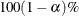confidence intervals

Computes confidence intervals for parameters

Computes confidence intervals for odds ratios

Options for Display of Details

Displays correlation matrix

Displays covariance matrix

Displays exponentiated values of estimates

Displays gradients evaluated at null hypothesis

Displays iteration history

Suppresses "Class Level Information" table

Displays parameter labels

Displays generalized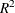Displays standardized estimates

The following list describes these options:

ABSFCONV=value

specifies the absolute function convergence criterion. Convergence requires a small change in the log-likelihood function in subsequent iterations: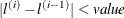where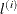is the value of the log-likelihood function at iteration i. See the section Convergence Criteria.

ALPHA=value

sets the level of significancefor% confidence intervals for regression parameters or odds ratios. The valuemust be between 0 and 1. By default,is equal to the value of the ALPHA= option in the PROC SURVEYLOGISTIC statement, or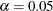if the ALPHA= option is not specified. This option has no effect unless confidence intervals for the parameters or odds ratios are requested.

CLODDS

requests confidence intervals for the odds ratios. Computation of these confidence intervals is based on individual t tests or Wald tests. The degrees of freedom for a t test degrees of freedom is described in the section Degrees of Freedom. The confidence coefficient can be specified with the ALPHA= option. See the section Wald Confidence Intervals for Parameters for more information.

CLPARM

requests confidence intervals for the parameters. Computation of these confidence intervals is based on the t tests or Wald tests. The degrees of freedom for a t test is described in the section Degrees of Freedom. The confidence level can be specified with the ALPHA= .

CORRB

displays the correlation matrix of the parameter estimates.

COVB

displays the covariance matrix of the parameter estimates.

DF=value | type

specifies the denominator degrees of freedom (df) for F statistics in hypothesis testing, and the degrees of freedom in t tests for parameter estimates, odds ratio estimates, and their t percentiles for confidence limits. You can specify DF=value, where value is a nonnegative number, or you can specify DF=type, where type can be DESIGN, INFINITY, or PARMADJ.

If you specify both this option and the DF= option in a REPWEIGHTS statement, PROC SURVEYLOGISTIC uses this option to determine the df.

You can specify one of the following types:

DESIGN

determines the df from the survey design and the variance estimation method. For more information, see the section Degrees of Freedom. When you specify this option, PROC SURVEYLOGISTIC determines the value of df as follows.

• For Taylor series variance estimation, df is calculated as follows:

• the number of clusters minus the number of strata if the design is stratified and has clusters

• the number of clusters minus 1 if the design has clusters and is not stratified

• the total sample size minus the number of strata if the design is stratified and has no clusters

• the total sample size minus the number of strata if the design is not stratified and has no clusters

• If you provide replicate weights (in the REPWEIGHTS statement), df is the number of replicates. Alternatively, you can use the DF= option in a REPWEIGHTS statement to specify the value of df.

• For BRR (including Fay’s method ) variance estimation (when you do not specify a REPWEIGHTS statement), df is the number of strata.

• For jackknife variance estimation (when you do not specify a REPWEIGHTS statement), PROC SURVEYLOGISTIC computes df as the number of replicates minus the number of strata. If the design is not stratified, df is the number of replicates minus one.

INFINITY
NONE

specifies that the df is infinite. When the denominator degrees of freedom for an F test is infinite, the F tests is equivalent to a chi-square test. When the degrees of freedom for a t percentile is infinite, the t percentile is equivalent to a normal percentile. Therefore, when you specify DF=INFINITY, PROC SURVEYLOGISTIC uses chi-square tests (instead of F tests) and normal percentiles (instead of t percentiles).

modifies the df by the number of nonsingular parameters in the model. This option applies only when the Taylor variance estimation method is used (either by default or by specifying VARMETHOD=TAYLOR ). This option can be useful when you are fitting a model that has many parameters relative to the default degrees of freedom. See the section Degrees of Freedom for more information.

By default, DF=DESIGN. For more information, see the section Degrees of Freedom.

EXPB
EXPEST

displays the exponentiated values (e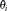) of the parameter estimates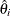in the "Analysis of Maximum Likelihood Estimates" table for the logit model. These exponentiated values are the estimated odds ratios for the parameters corresponding to the continuous explanatory variables.

FCONV=value

specifies the relative function convergence criterion. Convergence requires a small relative change in the log-likelihood function in subsequent iterations: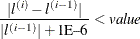whereis the value of the log likelihood at iteration i. See the section Convergence Criteria for details.

GCONV=value

specifies the relative gradient convergence criterion. Convergence requires that the normalized prediction function reduction is small: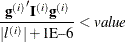whereis the value of the log-likelihood function,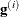is the gradient vector, and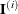the (expected) information matrix. All of these functions are evaluated at iteration i. This is the default convergence criterion, and the default value is 1E–8. For more information, see the section Convergence Criteria.

displays the gradient vector, which is evaluated at the global null hypothesis.

ITPRINT

displays the iteration history of the maximum-likelihood model fitting. The ITPRINT option also displays the last evaluation of the gradient vector and the final change in the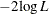.

L=keyword

specifies the link function that links the response probabilities to the linear predictors. You can specify one of the following keywords. The default is LINK=LOGIT.

CLOGLOG

specifies the complementary log-log function. PROC SURVEYLOGISTIC fits the binary complementary log-log model for binary response and fits the cumulative complementary log-log model when there are more than two response categories. Aliases: CCLOGLOG, CCLL, CUMCLOGLOG.

GLOGIT

specifies the generalized logit function. PROC SURVEYLOGISTIC fits the generalized logit model where each nonreference category is contrasted with the reference category. You can use the response variable option REF= to specify the reference category.

LOGIT

specifies the cumulative logit function. PROC SURVEYLOGISTIC fits the binary logit model when there are two response categories and fits the cumulative logit model when there are more than two response categories. Aliases: CLOGIT, CUMLOGIT.

PROBIT

specifies the inverse standard normal distribution function. PROC SURVEYLOGISTIC fits the binary probit model when there are two response categories and fits the cumulative probit model when there are more than two response categories. Aliases: NORMIT, CPROBIT, CUMPROBIT.

See the section Link Functions and the Corresponding Distributions for details.

MAXITER=n

specifies the maximum number of iterations to perform. By default, MAXITER=25. If convergence is not attained in n iterations, the displayed output created by the procedure contains results that are based on the last maximum likelihood iteration.

NOCHECK

disables the checking process to determine whether maximum likelihood estimates of the regression parameters exist. If you are sure that the estimates are finite, this option can reduce the execution time when the estimation takes more than eight iterations. For more information, see the section Existence of Maximum Likelihood Estimates.

NODUMMYPRINT

suppresses the "Class Level Information" table, which shows how the design matrix columns for the CLASS variables are coded.

NOINT

suppresses the intercept for the binary response model or the first intercept for the ordinal response model.

OFFSET=name

names the offset variable. The regression coefficient for this variable is fixed at 1.

PARMLABEL

displays the labels of the parameters in the "Analysis of Maximum Likelihood Estimates" table.

RIDGING=ABSOLUTE | RELATIVE | NONE

specifies the technique used to improve the log-likelihood function when its value in the current iteration is less than that in the previous iteration. If you specify the RIDGING=ABSOLUTE option, the diagonal elements of the negative (expected) Hessian are inflated by adding the ridge value. If you specify the RIDGING=RELATIVE option, the diagonal elements are inflated by a factor of 1 plus the ridge value. If you specify the RIDGING=NONE option, the crude line search method of taking half a step is used instead of ridging. By default, RIDGING=RELATIVE.

RSQUARE

requests a generalizedmeasure for the fitted model.

SINGULAR=value

specifies the tolerance for testing the singularity of the Hessian matrix (Newton-Raphson algorithm) or the expected value of the Hessian matrix (Fisher scoring algorithm). The Hessian matrix is the matrix of second partial derivatives of the log likelihood. The test requires that a pivot for sweeping this matrix be at least this value times a norm of the matrix. Values of the SINGULAR= option must be numeric. By default, SINGULAR=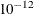.

STB

displays the standardized estimates for the parameters for the continuous explanatory variables in the "Analysis of Maximum Likelihood Estimates" table. The standardized estimate of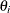is given by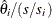, where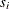is the total sample standard deviation for the ith explanatory variable and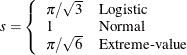For the intercept parameters and parameters associated with a CLASS variable, the standardized estimates are set to missing.

TECHNIQUE=FISHER | NEWTON
TECH=FISHER | NEWTON

specifies the optimization technique for estimating the regression parameters. NEWTON (or NR) is the Newton-Raphson algorithm and FISHER (or FS) is the Fisher scoring algorithm. Both techniques yield the same estimates, but the estimated covariance matrices are slightly different except for the case where the LOGIT link is specified for binary response data. The default is TECHNIQUE=FISHER. If the LINK=GLOGIT option is specified, then Newton-Raphson is the default and only available method. See the section Iterative Algorithms for Model Fitting for details.

VADJUST=DF | MOREL <(Morel-options)> | NONE

specifies an adjustment to the variance estimation for the regression coefficients.

If you do not want to use any variance adjustment, you can specify the VADJUST=NONE option. You can specify the VADJUST=MOREL option for the variance adjustment proposed by Morel (1989).

You can specify the following Morel-options within parentheses after the VADJUST=MOREL option:

ADJBOUND=sets the upper bound coefficientin the variance adjustment. This upper bound must be positive. By default, the procedure uses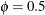. See the section Adjustments to the Variance Estimation for more details on how this upper bound is used in the variance estimation.

DEFFBOUND=
sets the lower bound of the estimated design effect in the variance adjustment. This lower bound must be positive. By default, the procedure uses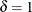. See the section Adjustments to the Variance Estimation for more details about how this lower bound is used in the variance estimation.

XCONV=value

specifies the relative parameter convergence criterion. Convergence requires a small relative parameter change in subsequent iterations: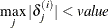where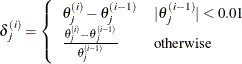and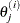is the estimate of the jth parameter at iteration i. See the section Convergence Criteria for details.